# Kinetics Activity

-Introduction

-Background Step 1

-Background Step 2

-Step 1: Making a kinetic trace

-Step 2a: Getting information from a kinetic trace

-Step 2b: Determining the pseudo rate constant k'

-Step 3: Determine the effect of bleach on the reaction

-Step 4: Converting absorbance to concentration

Kinetics Studies of the Bleaching of Food Dyes >> Background Step 2

 < Previous | Next >

# Kinetics Studies of the Bleaching of Food Dyes

## Finding the order of the reaction with respect to dye.

We will consider three possible orders for the reaction:which may be simplified to: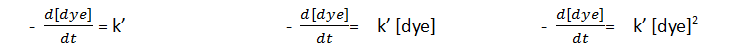Integrating these differential equations gives the dye concentration as a function of time: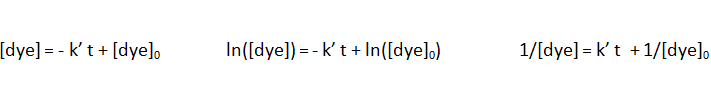The following plots show how [dye] changes with time for each of these cases.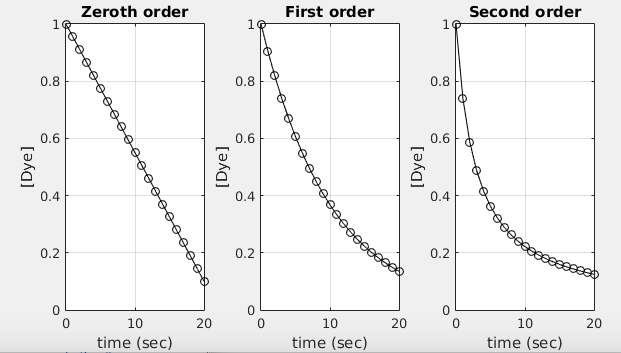For zeroth-order reaction, the rate of the reaction is independent of dye concentration, so we get a straight line with slope k'.

For a first-order reaction, the rate is proportional to [dye], so the rate decreases as the reaction proceeds and [dye] decreases.

For a second-order reaction, the rate is proportional to [dye]2, and the decrease in rate as the reaction proceeds is more rapid than for the first order reaction.

A useful technique for identifying first order reactions is to plot ln([dye]) versus time: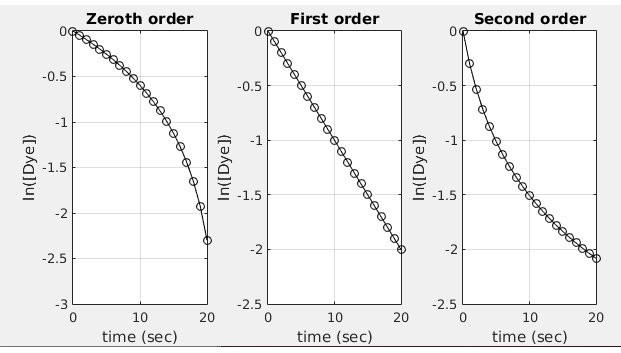Note that only the first order reaction gives a straight line.

If we plot 1/[dye] versus time, only the second order reaction gives a straight line:This leads to a useful means to identify the order of reaction. Given a kinetic trace, we compare the following three plots: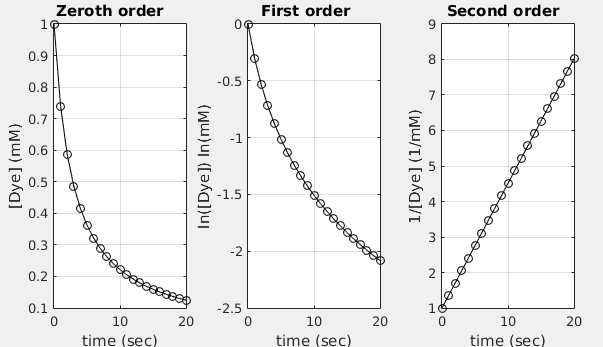For this data, the plot of 1/[dye] versus time gives a straight line. This indicates that the reaction is a second order reaction and the rate law is: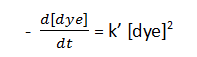Suppose the kinetic trace you measured in the lab led to the following three plots: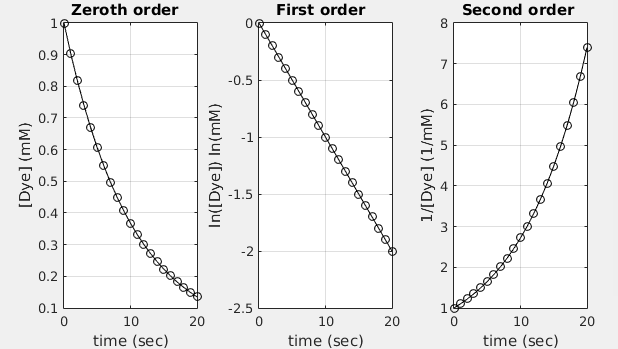Which of the following is the rate law for the reaction?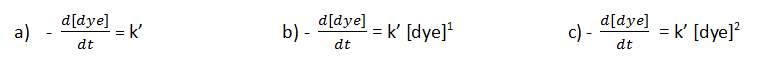The description above explains how to use this plotting technique to determine the order of a reaction. To understand why it works, please see the explanation of "integrated rate laws" in the textbook and your class notes.

 < Previous | Next >
 Contact Us Last Updated: Thursday, March 10th, 2022 @ 05:15:38 am